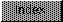```

NAME
IEEESPTst -- compare IEEE single precision value to 0.0

SYNOPSIS
c   = IEEESPTst(  y  );
d0                d0

float   y;
long    c;

FUNCTION
Compare y to 0.0, set the condition codes for less than, greater
than, or equal to 0.0.  Set the return value c to -1 if less than,
to +1 if greater than, or 0 if equal to 0.0.

INPUTS
y -- IEEE single precision floating point value

RESULT
c = 1   cc = gt         for (y > 0.0)
c = 0   cc = eq         for (y == 0.0)
c = -1  cc = lt         for (y < 0.0)

BUGS